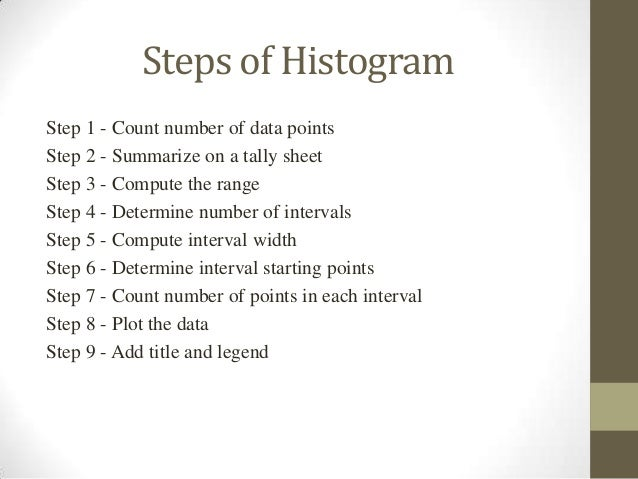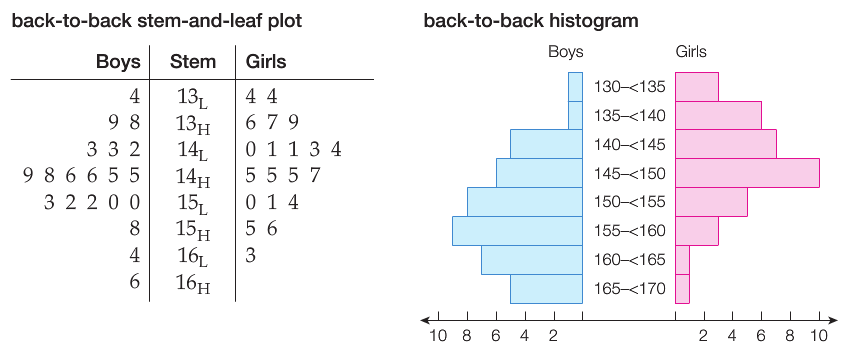# Statistics

histogramHistograms

year 9

further maths chapter 11. Find the maximum frequency and draw the vertical (y–axis) from zero to this value, including a sensible numeric scale.

2. The range of the horizontal (x–axis) needs to include not only the full range of observations but also the full range of the class intervals from the frequency table.

3. Draw a bar for each group in your frequency table. These should be the same width and touch each other (unless there are no data in one particular class).Outliers Calculation

Outliers Calculation

1. Step 1: Arrange the numbers in order.
2, 5, 6, 7, 8, 15
2. Step 2: determine the midpoint number or median.
2, 5, 6, 7, 8, 15
6 + 7 = 13 ÷ 2 = 5
3. Step 3: calculate the median of the lower or first quartile (Q1)
2, 5, 6    Q15
4. Step 4: calculate the median of the upper or third quartile (Q3)
7, 8, 9    Q38
5. Step 5: calculate the interquartile (IQR)by subtracting (Q3) – (Q1)
8 – 5 = 3    (IQR) = 3
6. Step 6: multiply the (IQR) by 1.5
(IQR) × 1.5 = 3 × 1.5 = 5
7. Step 7: calculate the lower outlier range by subtracting (Q1) minus step 6
(Q1) – (IQR× 1.5) = 5 – 4.5 = 5
the lower outlier range is any numbers 1.5 or lower
8. Step 8: calculate the upper outlier range by adding (Q3) plus step 6
(Q3) + (IQR× 1.5) = 8 + 4.5 = 5
the upper outlier range is any numbers 12.5 or higher

Q7 b (page 22)

Step 1

10,14,8,12,11,16,3,18,11,15,9,20,4,17,9,22,10,15,9,14

Arrange the data in order

3, 4, 8, 9, 9, 9, 10, 10, 11, 11, 12, 14, 14,15, 15, 16, 17, 18, 20, 22 { 20 data values}

STEP 2

Since n =20,      = = 10.5

Therefore the median =     =       =  11.5

Step 3

Since the median is not a data value, we split the original data into two equal groups of 10

3, 4, 8, 9, 9, 9, 10, 10, 11, 11             12, 14, 14,15, 15, 16, 17, 18, 20, 22

Q1 = 9                                                     Q3 = 15.5

IQR = 15.5 – 9

IQR = 6.5

= 1.5  X 6.5

= 9.75

# Q3+1.5 X IQR = 15.5 + 9.75 = 25.25

A data point is an outlier if it is either less

Q1 -1.5 X  IQR    OR

Greater than                         Q3 +1.5 X  IQR

A data point is an outlier if it is either less

Q1 -1.5 X  IQR    OR

Greater than                         Q3 +1.5 X  IQR

SSD